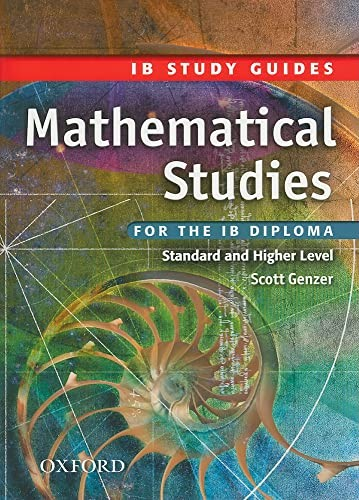Free Shipping in Australia# IB Study Guide: Mathematical Studies by Scott Genzer

Used Price
\$23.49
inc. GST
Free Shipping in Australia

Only 3 left

## IB Study Guide: Mathematical Studies Summary

### IB Study Guide: Mathematical Studies: For the IB Diploma by Scott Genzer

This study guide will help students further understand basic concepts and will reinforce concepts already learned through excellent examples. With a wealth of questions from past IB exam papers, three completely new IB-style exams, graphing calculator help and test-taking advice from teachers and students, this book will help students thoroughly prepare for the exam.

## Customer Reviews - IB Study Guide: Mathematical Studies

### Why buy from World of BooksOur excellent value books literally don't cost the earthFree delivery in AustraliaEvery used book bought is one saved from landfill

INTRODUCTION; STRUCTURE OF THE IB MATHEMATICAL STUDIES SL EXAM; SCORING OF THE IB MATHEMATICAL STUDIES SL EXAM; HINTS FROM IRENE, THE IB EXAMINER; HINTS FROM HANA AND CHRIS, THE IB STUDENTS; HINTS FROM SCOTT, THE IB TEACHER; TOPICS OF THE SYLLABUS; NUMBER AND ALGEBRA; 1. Sequences and series - arithmetic; 2. Sequences and series - geometric; 3. Number sets; 4. Approximation, significant figures, percentage errors, estimation; 5. Scientific notation and the SI (metric) system; 6. Word problems and systems of linear equations; 7. Quadratic equations; 8. Additional practice problems; SETS, LOGIC AND PROBABILITY; 1. Set theory and Venn diagrams; 2. Logic symbols and statements; 3. Truth Tables; 4. Probability: harder problems (compound and conditional probability); 5. Additional practice problems; FUNCTIONS; 1. Definition of a function, mapping diagrams, domain and range; 2. Linear functions and graphs; 3. Quadratic functions and graphs; 4. Exponential functions and graphs; 5. Trigonometric functions and graphs; 6. Unfamiliar functions and graphs; 7. Additional practice problems; GEOMETRY AND TRIGONOMETRY; 1. Coordinates, midpoint and distant formulae; 2. Equation of a line: forms, slope/gradient, perpendicular and parallel lines; 3. Right-angled triangle trigonometry (SOHCAHTOA); 4. Sine rule and area of a triangle; 5. Cosine rule; 6. Geometry of 3-D shapes; 7. Additional practice problems; STATISTICS; 1. Classification of date, frequency tables and polygons; 2. Grouped data, histograms, stem-and-leaf plots; 3. Cumulative frequencies, box-and-whisker plots; 4. 1-variable statistical calculations; 5. Linear regression (line of best fit); 6. Chi-squared independent test; 7. Additional practice problems; INTRODUCTORY DIFFERENTIAL CALCULUS; 1. Differentiation and derivative of a polynomial; 2. Equations of tangent lines, values of x when f is given; 3. Increasing and decreasing functions, max/min problems (optimisation problems); 4. Additional practice problems; FINANCIAL MATHEMATICS; 1. Currency conversion; 2. Simple and compound interest; 3. Loan and savings tables; 4. Additional Practice problems; PRACTICE EXAM; IB MATHEMATICAL STUDIES SL FORMULA BOOKLET

GOR004320382
IB Study Guide: Mathematical Studies: For the IB Diploma by Scott Genzer
Scott Genzer
Used - Very Good
Paperback
Oxford University Press
2009-03-26
208
019915242X
9780199152421
N/A
Book picture is for illustrative purposes only, actual binding, cover or edition may vary.
This is a used book - there is no escaping the fact it has been read by someone else and it will show signs of wear and previous use. Overall we expect it to be in very good condition, but if you are not entirely satisfied please get in touch with us.